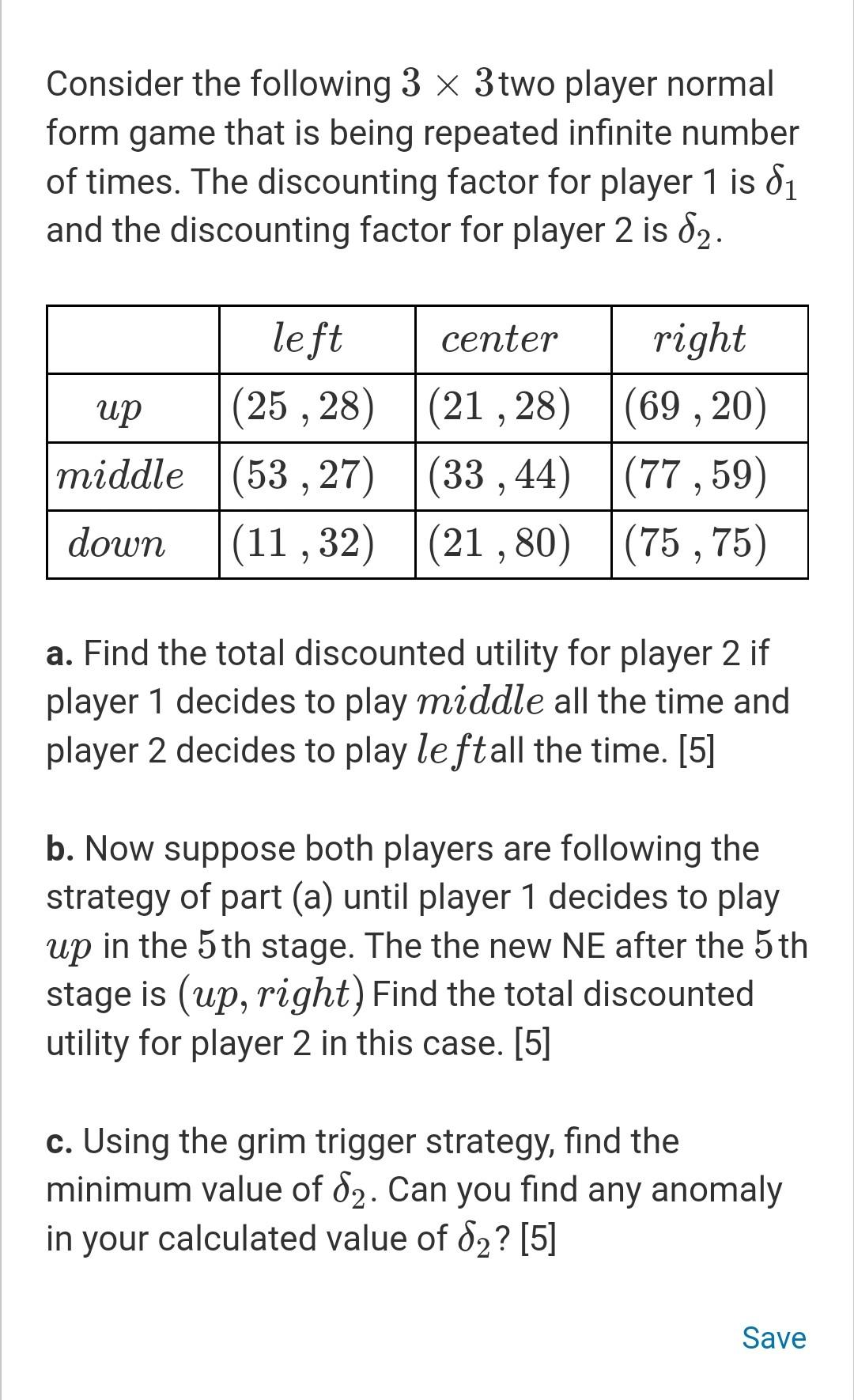# (Solved): i would really appreciate if you would help me. thanks in advance   Consider the followi ...

i would really appreciate if you would help me.Consider the following $$3 \times 3$$ two player normal form game that is being repeated infinite number of times. The discounting factor for player 1 is $$\delta_{1}$$ and the discounting factor for player 2 is $$\delta_{2}$$. a. Find the total discounted utility for player 2 if player 1 decides to play middle all the time and player 2 decides to play le ftall the time.  b. Now suppose both players are following the strategy of part (a) until player 1 decides to play $$u p$$ in the 5 th stage. The the new NE after the 5 th stage is (up,right) Find the total discounted utility for player 2 in this case.  c. Using the grim trigger strategy, find the minimum value of $$\delta_{2}$$. Can you find any anomaly in your calculated value of $$\delta_{2}$$ ? 

We have an Answer from Expert